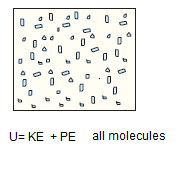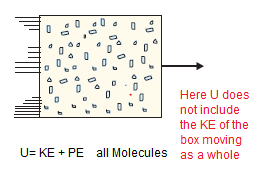# Internal Energy

## Internal Energy

• Internal energy can be described as the sum of kinetic and potential energies of individual molecules in the system in the frame of reference relative to which the Center of mass of the system is at rest.
• But in thermodynamics one should keep in mind that U is simply a macroscopic variable of the system.
• U is thermodynamic state variable and its value depends only on the given state of the system and not on path taken to arrive the state.
• If we neglect the intermolecular forces between the molecules in the gas, the Internal energy of the gas is the sum of the Kinetic energy of the molecules which are moving in random motion. This will include translation kinetic energy and also rotational and vibrational kinetic energy of the molecules.
• So in short, Internal energy U of a gas is the sum of the kinetic and potential energies of its molecules when the box is at rest. Kinetic energy due to various types of motion (translational, rotational, vibrational) is to be included in U• Important point: If the same box is moving as a whole with some velocity, the kinetic energy of the box is not to be included in U• Transfer of heat and performance of work are two mean of adding or subtracting energy from a system.
• On transfer of energy, system is said to have undergone a change in internal energy.
• The Internal Energy of the given mass of gas depends on Pressure,Volume and temperature of the gas